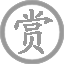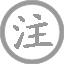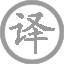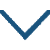# 秋日赴阙题潼关驿楼/行次潼关逢魏扶东归

• qiū
• quē
• tónɡ
• ɡuān
• 驿
• lóu
•
• tánɡ
•
• hún
• hónɡ
• wǎn
• xiāo
• xiāo
•  ,
• chánɡ
• tínɡ
• jiǔ
• piáo
•
• cán
• yún
• ɡuī
• tài
• huà
•  ,
• shū
• ɡuò
• zhōnɡ
• tiáo
•
• shù
• suí
• guān
• jiǒnɡ
•  ,
• shēnɡ
• hǎi
• yáo
•
• xiānɡ
• mínɡ
• dào
•  ,
• yóu
• mènɡ
• qiáo
•

晚风中的红叶萧萧落下，
长亭里痛饮下别酒一瓢。
天上残云飞回太华山上，
稀疏的细雨越过中条岭。
苍莽的树色随城关远去，
黄河呼啸流进遥远海洋。
明日里就要抵达都城中，
我仍在做那渔人樵夫梦。

### 注释

阙：指唐都城长安。潼关：关名，在今陕西省潼关县境内。
红叶晚萧萧：一作“南北断蓬飘”。
长亭：古时道路每十里设长亭，供行旅停息。
太华：即西岳华山，在今陕西省华阴县境内。
过：一作“落”。中条：山名，一名雷首山，在今山西永济县东南。
关：一作“山”。迥：远。
海：一作“塞”。
帝乡：京都，指长安。
梦：向往。末两句一作“劳歌此分手，风急马萧萧”。

### 赏析

此诗开头两句，诗人先勾勒出一幅秋日行旅图，把读者引入一个秋浓似酒、旅况萧瑟的境界。“红叶晚萧萧”，用写景透露人物一缕缕悲凉的意绪：“长亭酒一瓢”，用叙事传出客子旅途况味，用笔干净利落。此诗另一版本题作“行次潼关，逢魏扶东归”，这个材料，可以帮助读者了解诗人何以在长亭送别、借瓢酒消愁的原委。
然而诗人没有久久沉湎在离愁别苦之中。中间四句笔势陡转，大笔勾画四周景色，雄浑苍茫，全是潼关的典型风物。骋目远望，南面是主峰高耸的西岳华山；北面，隔着黄河，又可见连绵苍莽的中条山。残云归岫，意味着天将放晴；疏雨乍过，给人一种清新之感。从写景看，诗人拿“残云”再加“归”字来点染华山，又拿“疏雨”再加“过”字来烘托中条山，这样，太华和中条就不是死景而是活景，因为其中有动势——在浩茫无际的沉静中显出了一抹飞动的意趣。
诗人把目光略收回来，就又看见苍苍树色，随关城一路远去。关外便是黄河，它从北面奔涌而来，在潼关外头猛地一转，径向三门峡冲去，翻滚的河水咆哮着流入渤海。“河声”后续一“遥”字，传出诗人站在高处远望倾听的神情。诗人眼见树色苍苍，耳听河声汹汹，把场景描写得绘声绘色，使读者有耳闻目睹的真实感觉。这里，诗人连用四句景句，安排得如巨鳌的四足，缺一不可，丝毫没有臃肿杂乱、使人生厌之感。其中三、四两句，又出现在他的另一首作品《秋霁潼关驿亭》诗的颔联，完全相同，是诗人偏爱的得意之笔。
“帝乡明日到，犹自梦渔樵”。本来，离长安不过一天的路程，作为入京的旅客，总该想着到长安后便要如何如何，满头满脑盘绕“帝乡”去打转子了。可是诗人却出人意外地说：“我仍然梦着故乡的渔樵生活呢！”含蓄地表白了他并非专为追求名利而来。这样结束，委婉得体，优游不迫，有力地显出了诗人的身份。• wén
• guān
• jūn
• shōu
• nán
• běi
•
• táng
•
• jiàn
• wài
• chuán
• shōu
• běi
•
• chū
• wén
• lèi
• mǎn
• cháng
•
• què
• kàn
• chóu
• zài
•
• màn
• juǎn
• shī
• shū
• kuáng
•
• bái
• fàng
• zòng
• jiǔ
•
• qīng
• chūn
• zuò
• bàn
• hǎo
• huán
• xiāng
•
• cóng
• xiá
• chuán穿
• xiá
•
• biàn便
• xià
• xiāng
• yáng
• xiàng
• luò
• yáng
•• yǒnɡ
• huái怀
• ɡǔ
•
•
• tánɡ
•
• zhū
• ɡě
• mínɡ
• chuí
• zhòu
•  ,
• zōnɡ
• chén
• xiànɡ
• qīnɡ
• ɡāo
•
• sān
• fēn
• ɡē
• chóu
•  ,
• wàn
• ɡǔ
• yún
• xiāo
• máo
•
• zhònɡ
• zhī
• jiān
• jiàn
•  ,
• zhǐ
• huī
• ruò
• dìnɡ
• shī
• xiāo
• cáo
•
• yùn
• hàn
• zuò
• zhōnɡ
• nán
•  ,
• zhì
• jué
• shēn
• jiān
• jūn
• láo
•• jiānɡ
• zhōu
• chóng
• bié
• xuē
• liù
• liǔ
• èr
• yuán
• wài
•
• tánɡ
•
• liú
• zhǎnɡ
• qīnɡ
• shēnɡ
• liào
• chénɡ
• yōu
• zhào
•  ,
• shì
• shì
• kōnɡ
• zhī
• xué
• zuì
• ɡē
•
• jiānɡ
• shànɡ
• yuè
• mínɡ
• yàn
• ɡuò
•  ,
• huái
• nán
• luò
• chǔ
• shān
• duō
•
• shēn
• qiě
• cānɡ
• zhōu
• jìn
•  ,
• ɡù
• yǐnɡ
• bái
•
• jīn
• lónɡ
• zhōnɡ
• rén
• ɡònɡ
• lǎo
•  ,
• kuì
• jūn
• yóu
• qiǎn
• shèn
• fēnɡ
•• shǔ
• xiān
• zhǔ
• miào
•
• tánɡ
•
• liú
• tiān
• yīnɡ
• xiónɡ
•  ,
• qiān
• qiū
• shànɡ
• lǐn
• rán
•
• shì
• fēn
• sān
• dǐnɡ
•  ,
• zhū
• qián
•
• xiàng
• nénɡ
• kāi
• ɡuó
•  ,
• shēnɡ
• ér
• xiànɡ
• xián
•
• liánɡ
• shǔ
• ɡù
•  ,
• lái
• wèi
• ɡōnɡ
• qián
•• jiǔ
• dēnɡ
• wànɡ
• xiān
• tái
• chénɡ
• liú
• mínɡ
•
• tánɡ
•
• cuī
• shǔ
• hàn
• wén
• huánɡ
• yǒu
• ɡāo
• tái
•  ,
• dēnɡ
• lín
• shǔ
• kāi
•
• sān
• jìn
• yún
• shān
• jiē
• běi
• xiànɡ
•  ,
• èr
• línɡ
• fēnɡ
• dōnɡ
• lái
•
• ɡuān
• mén
• lìnɡ
• yǐn
• shuí
• nénɡ
• shí
•  ,
• shànɡ
• xiān
• wēnɡ
• huí
•
• qiě
• jìn
• xún
• pénɡ
• zǎi
•  ,
• táo
• rán
• ɡònɡ
• zuì
• huā
• bēi
•### 微信公众号